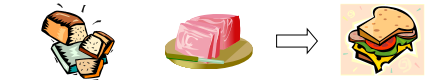## Lab 5 Introduction

### Learning Objectives

• Use stoichiometry to determine the limiting reactant.
• Calculate the theoretical yield.
• Calculate the percent yield of a reaction.

## Introduction

In lecture you have learned to read chemical equations and evaluate the mol to mol ratios of reactants and products involved in a chemical reaction. In laboratory experiments it is difficult to measure out chemicals in the exact ratio necessary for the chemical reaction. For time and speed reasons, the reaction mixtures in lab will usually have a limiting and an excess reactant.

Limiting reagent (also called limiting reactant) problems use stoichiometry to determine the theoretical yield for a chemical reaction. The limiting reactant will be completely consumed in the reaction and limits the amount of product you can make. The limiting reactant also determines the amount of product you can make (the theoretical yield). The reactant that is left over after the reaction is complete is called the excess reactant.

### Example 1

Consider the process it takes to make a ham sandwich. You need two slices of bread and one piece of ham to make each sandwich. How many complete sandwiches could you make if you had eighteen slices of bread and six slices of ham? Let’s set this up like a chemical equation where the ham and bread are our reactants and the sandwich is our product:

Two slices of bread    +      1 piece of ham =       1 ham sandwichNow we can use stoichiometry to determine the amount of sandwiches we could make if we used all of our reactants.

$\displaystyle18\text{ slices of bread}\frac{1\text{ sandwich}}{2 \text{ pieces of bread}}=9\text{ sandwiches}\\$

$\displaystyle6\text{ slices of bread}\frac{1\text{ sandwich}}{2 \text{ slice of ham}}=6\text{ sandwiches}\\$

We have enough bread to make 9 sandwiches. There is not enough ham available to make as many sandwiches. If we use all the ham, we can only make 6 sandwiches. Since the ham limits the number of sandwiches we can make, the ham is our limiting reagent and the bread is going to be in excess when the ham is consumed by the reaction. Additionally, since the theoretical yield depends on the limiting reactant, we can say that our theoretical yield for the above reaction is 6 sandwiches. It does not matter that there is enough bread to make 9 sandwiches. Once the ham runs out, it is not possible to make any more sandwiches. The reaction is complete at this point. Limiting reactant is completely consumed while the excess reactant (bread) is left over. We could even take this a step further and determine the amount of excess reagent left over at the end of the reaction. In order to perform that calculation, use the theoretical yield to calculate the amount of excess reactant used in the reaction. Then you can subtract the amount of excess reactant used in the chemical equation from the amount you began with.

### Example 2

For example given the balanced reaction $\text{N}_{2}+2\text{H}_{3}\rightarrow2\text{NH}_{3}$. If you began with 28 g of N2 and 2.8 g of H2. Since it is not possible to determine which reactant is the limiting reactant simply from the masses of the reactants, you must first convert the grams to moles using the molecular weights.

Therefore, $\displaystyle28\text{g N}_2=\frac{1\text{ mol N}_2}{28.0\text{ g N}_2}=1\text{ mole N}_2\\$

And $\displaystyle2.8\text{ g H}_2=\frac{1\text{ mol H}_2}{2.02\text{ g H}_2}=1.4\text{ mole H}_2\\$

While there is indeed more H2 than N2 based on moles of reactants, this is not the final answer! You must convert to the mol of product using the mol to mol ratio.

If N2 is completely used

1 mole of N2 $\displaystyle\frac{2\text{ mol NH}_3}{1\text{ mol N}_2}=\underline{2 \text{mol NH}_{3}}\\$ produced

If H2 is completely used

1.4 mole of H2 $\displaystyle\frac{2\text{ mol NH}_3}{3\text{ mol H}_2}=\underline{0.93\text{ mol NH}_{3}}\\$ produced

Thus, since H2 will produce less of the product, it is the limiting reagent and N2 is the excess reagent. Here the theoretical yield is 0.93 mol NH3. Note that for the problems in today’s lab we will then convert the mol of product to grams using its molar mass.

### Percent Yield

It is often important to calculate the percent yield of a reaction. If everything goes according to plan, you will get exactly 100 percent of the theoretical yield produced in your reaction. However, laboratory errors will often affect this number. Spills, calculation errors, not drying a product and many other errors affect the mass of product obtained. Here the amount of product actually produced in the laboratory experiment is compared to the amount of product that should have been made theoretically. Percent yield is given by the equation:

$\displaystyle\text{Percent Yield}=\frac{\text{Actual (Experimental) Yield}}{\text{Theoretical Yield}}\times100\\$

Guidelines for Limiting Reagent Problems (Calculating Theoretical Yield):

• Convert from grams of reactant added to mol using molar mass.
• Convert from mol of reactant to mol of product using the coefficients in the balanced equation (mol to mol ratio).
• Convert from mol of product to mol of reactant using the molar mass.

$2\text{ Al(s)}+3\text{ CuCl}_{2}\cdot2\text{H}_{2}\text{O}(aq)\rightarrow3\text{ Cu (s)}+2\text{ AlCl}_{3}(aq)+6\text{ H}_{2}\text{O(l)}$
*Note that the hydrate portion of $\text{CuCl}_{2}\cdot2\text{ H}_{2}\text{O}$ should be included in the molar mass calculation.